# 3. Pair Trading, Method 1, Chapter 1 (PTM1, C1) -Tracking Pairs

## 3.1 – Getting you familiar with Jargons

Like I had mentioned in the previous chapter, there are two techniques based on which you can pair trade. The first technique that we will discuss starting now, is usually referred to as the correlation based technique. I consider this as a fairly standard approach as many traders get their pair trading handholding of sorts using this approach.We need to learn few jargons before we get started on the actual technique, so let’s get to that straight. The jargons we will talk about in this chapter are related to tracking pairs. At this stage, I just want you to know what is what. We will connect the dots as we proceed.

Spreads – The spread, is perhaps the most versatile jargon used in the trading world. For example, if you are scalping the market then the word spread refers to the Rupee differential between the bid price and the ask price. Now, if you are doing an arbitrage trade, then the word spread refers to the difference between the prices of the same asset across two different markets. In the pair trading world (actually, just correlation-based technique), the word spread refers to the difference between the closing prices of two stocks.

The spread is calculated as –

Spread = Closing value of stock 1 – closing value of stock 2

Take a look at this –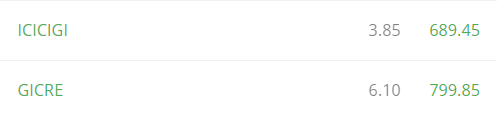If I assume GICRE as a stock 1 and ICICIGI as stock 2, then the spread is calculated as –

= 2.25

Please note, both 6.1 and 3.85 represents a change in stock price with respect to the previous close. Also, both the numbers are positive here. Now, for a moment assume, the closing price of ICICIGI was negative 3.85, in this case, the spread would turn out to be –

6.1-(-3.85)

= 9.95

I’ve calculated the spread for the last couple of trading days, this should give you an idea of how the spread ‘runs’. Also, since I’ve calculated the spread on a daily basis, traders refer to this as the ‘historical spread’.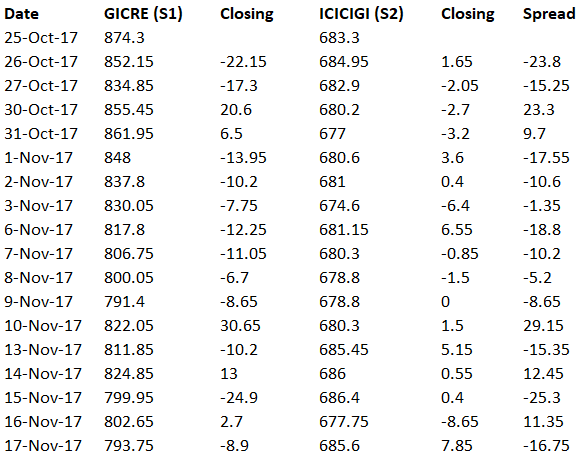As you can see, the spread varies on a daily basis. Also, here is an interesting (general) observation –

1. The spread expands if the closing value of S1 is positive and S2 is negative
2. The spread contracts if the closing value of S1 is positive and S2 is also positive

Of course, there are other possible combinations which lead to the expansion of contraction of the spreads. More on this later.

Differential – Unlike spreads, the differential measures the difference in the stock prices. The differential measures the absolute difference in the closing stock prices of two stock. The formula is as below –

Differential = Closing Price of Stock 1 – Closing Price of Stock 2

So if a stock 1 has closed at Rs.175 and stock 2 has closed at 232, the differential is –

175 – 232

= – 57

As you may have guessed, you can run this as a time series and calculate this on a daily basis, I’ve done this for GICRE and ICICIGI –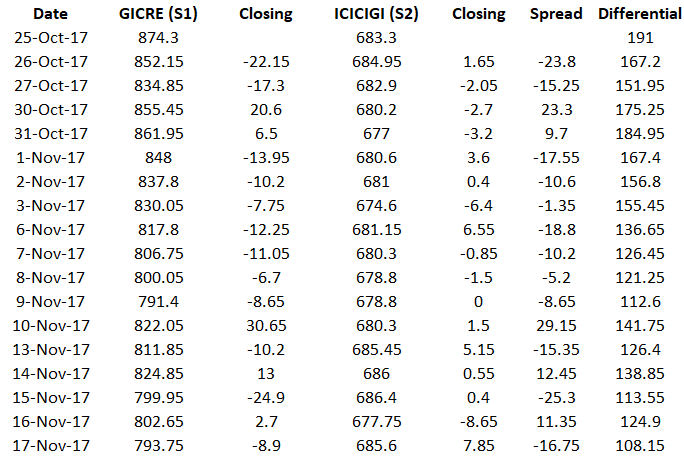Here is something you need to know about differentials – if you are using spreads to track pairs, then you can use it on an intraday basis. But unlike spreads, the ‘differentials’ is not a great technique to track pairs on an intraday basis, its best used at an end of day basis.

Of course, more on these things later. For now, let’s just focus on busting some jargons.

Ratio – I find the ratio bit quite interesting. The ratio is essentially dividing the stock price of stock 1 over the price of stock 2. Or it can be the other way round as well.

Ratio = Stock Price of stock 1 / stock price of stock 2

I’ve calculated the ratio of the same two stocks, here is how it looks –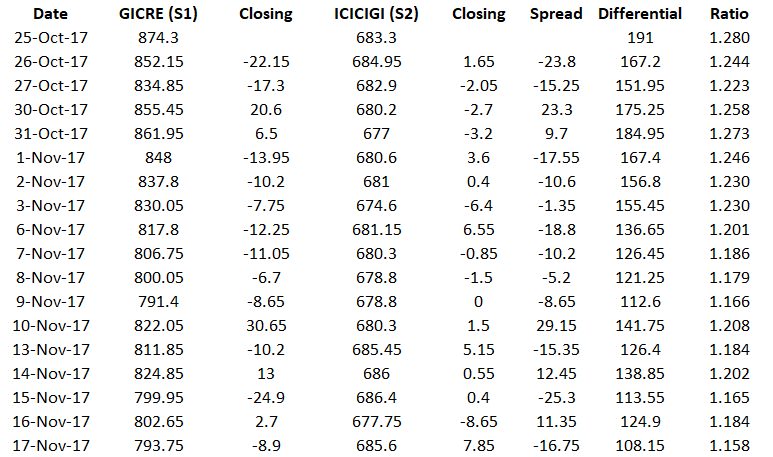The Ratio as you can see is a bit more consistent (or at least appears) when calculated as a time series. I’ve represented all the three variables on graph –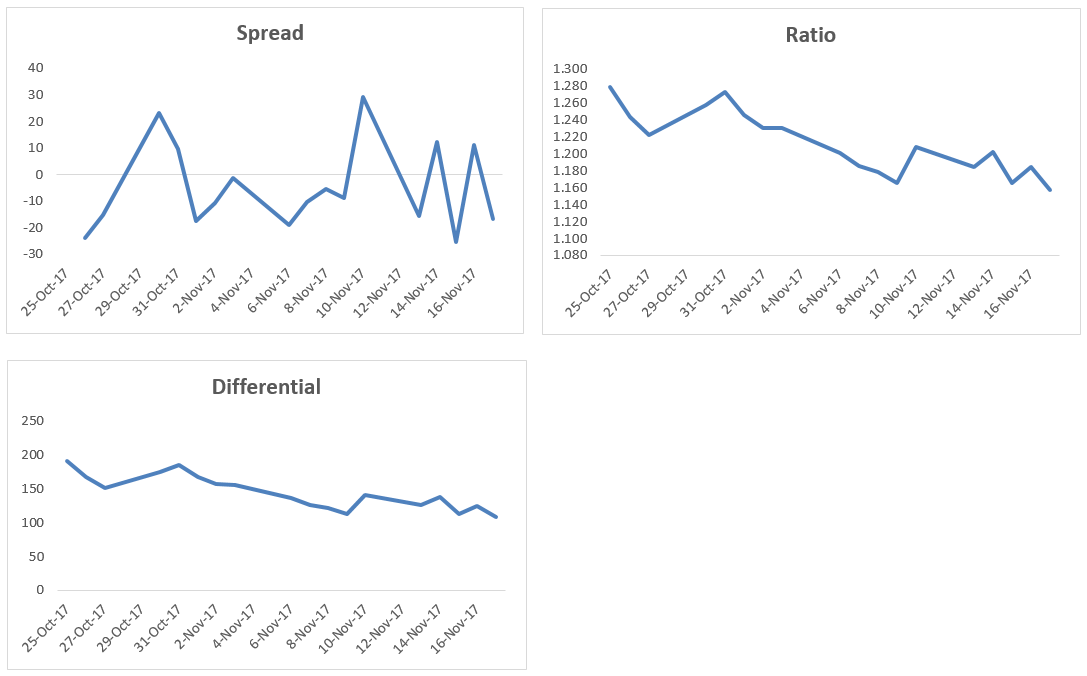So what are these things that we just looked at – spread, differential, and ratios and how are they related to pair trading?

Well, as you can imagine, these are the different variable which helps us measure or quantify the relationship between two stocks, which we consider as pairs. The graph tells us how the two stocks move with respect to each other. For instance, if we consider the spread, we know it expands if the closing value of S1 is positive and S2 is negative and the spread contracts if the closing value of S1 is positive and S2 is also positive.

Likewise in the ratio – the ratio between two stocks decrease if the stock prices of both the stock decline and the ratio increases if the stock prices of both the stocks increases. Of course, there are other variations possible – for example, the ratio can increase if stock 1 declines heavily and stock 2 stays flat or the other way round. Alternatively, stock 2 can increase a lot more compared to stock 1 or the other way round J

Confusing isn’t it?

Hence, for this reason, we need to look at the chart of the variable we are following, the variable could be spread, differential, or the ratio. We need to track the movement of the variable and figure out if the spread is expanding or contracting. This leads us to the next two jargons.

Divergence – If the ratio or the spread between the two stocks is expected to move apart or alternatively, you expect the graph to move up, then this translates to something called a divergence. When you expect your variable to diverge, you can make money (or at least attempt to make) by setting up a divergence trade.

Convergence – If the ratio or the spread between the two stocks is expected to move closer or alternatively, you expect the graph to move down, then this translates to something called as a convergence. When you expect your variable to converge, you can make money (or at least attempt to make) by setting up a convergence trade.

Now here is the big question – what makes you believe the variable can either converge or diverge? When do you decide to set up a trade? What are the triggers? How do you set up a trade? What if the trade does not work out? What is the stop-loss for such trades?

Well, even before we answer these questions, how do we qualify two stocks as a pair? Just because two stocks belong to the same sector, does that mean they qualify as a pair? For instance, does ICICI Bank and HDFC Bank qualify as a pair because they both belong to private sector banking?

To qualify two stocks as a pair we need to rely upon the good old statistical measure, called the ‘Correlation’. I guess, we have discussed correlation multiple times on varsity. Here is a quick explanation –

Correlation between two variables gives us a sense of how two variables move with respect to each other. Correlation is measured as a number which varies between -1 to +1. For example, if the correlation between two stocks is +0.75, then it tells us two things –

1. The plus preceding the number tells us that they both are positively correlated i.e when they move in the same direction
2. The actual number gives us a sense of the strength of this movement. In a loose sense, the closer it is to +1 (or -1) the higher is the tendency for the two variable to move in tandem.
3. A correlation of 0 suggests that the two variables are not related to each other.

From the above, we know a correlation of +0.75 suggests that the two variables move not only in the same direction but also tend to move together closely. Note, the correlation does not suggest the extent of the move, all it suggests is that the move in the same direction is likely to happen. For example, if Stock A moves 3%, and the correlation between stock A and stock B is +0.75, then it does not mean that Stock B will also move by 3%, all that the correlation suggests is that Stock B will move up positively, just like Stock A.

But, there is another twist here – suppose stock A and Stock B are correlated at 0.75, and the daily average return on Stock A and Stock B is 0.9% a 1.2%, then it can be said that on any given day, if Stock A moves above its daily average return of 0.9%, then stock B is also likely to move higher than its daily average return of 1.2%.

Likewise, a correlation of -0.75 indicates that the two variables move in opposite direction (-ve sign) but they both tend to move in opposite direction. Suppose stock A moves up by +2.5%, then by virtue of correlation we know that Stock B is likely to come down, but by what degree will it come down will not be known.

While we are at it, one more point on correlation. This bit is only for those interested in the math part of correlation. The correlation data makes sense only if the data series is ‘stationary around the mean’. What does this mean? – Well, it simply means that the data set should be sticking close the average values.

Keep this line ‘stationary around the mean’ in the back of your mind, don’t forget it. This will come back to again, when we discuss the 2nd technique to pair trade, much later in this module.

We will proceed with correlation as a measure to understand how tightly two stocks are coupled. In the next chapter, we will figure out how to calculate two different varieties of correlations.

For now, I want you to be clear on Spread, Differentials, Ratios, Divergence Trading, Convergence Trading, and Correlations!

### Key takeaways from this chapter

1. Spread measures the difference between the closing values of two stocks
2. Differentials measures the difference between the closing prices of two stock
3. Ratio between the two stocks essentially requires you to divide stock 1 over stock 2
4. Divergence is when you expect the two stocks to move apart
5. Convergence is when you expect the two stocks closer to each other
6. Correlation is like a glue which tells how tightly two stocks move together. ­­

1.Sujeet Raj says:

Things are getting interesting. Thank you!!!

Any approximation on the number of chapters you are planning for this module?

•Senpai says:

69

•Karthik Rangappa says:

I plan to introduce two different technique to pair trade. I’m guessing each technique will have around 6-8 chapters.

2.KUMAR MAYANK says:

Hello sir
As i got it:
Convergence= i) spread approaches zero (from both the sides ie -ve and +ve) ii) ratio tains to one. iii) graph of spread moves towards y(vertical axis)=0 from y= -ve ie upward and from y= +ve to y=0 ie downward.
Am i correct?
Thank you

•Karthik Rangappa says:

Yes, Kumar. More on this in the next chapter 🙂

•KUMAR MAYANK says:

I’m eagerly waiting as usual.
Thank you sir!

•Karthik Rangappa says:

3.NARASIMHA says:

Since zerodha is not allowing all stocks for intraday(eventhough they are eligible for intraday),Can we use cnc order type with full margin and square it off on the same day?Will it consider as intraday or stt charges are more???

•Faisal Mohammed says:

Yes, you can use CNC order type and take long(buy) intraday positions and square it off the same day except for Trade to Trade segment stocks. However, you cannot take short positions due to liquidity risk(Read about auction penalties on short delivery here).
Brokerage, STT and other charges will be charged on intraday basis only

•NARASIMHA says:

THANK you faisal sir.I hope zerodha will provide intraday mis order type facility to all stocks.I am loosing opportunities due to this issue.Feeling very sad to say that Iam changing from zerodha…:(

•NARASIMHA says:

Please give me the list of all t2t stocks sir.I want full list.

4.Sashidhar. L says:

Sir I’m very excited to see this module live. When you say pairs tracking can be used on intraday basis what does it mean? And while we are on the subject, can you suggest one good book on intraday trading techniques? Thanks a ton.

•Karthik Rangappa says:

It means tracking the variable’s movement on an intraday basis. The variable can be a spread or a ratio, or anything else that we will learn in the future chapters 🙂

•Sashidhar. L says:

Sir what about the book recommendation?

•Karthik Rangappa says:

Regarding?

•Sashidhar. L says:

•Karthik Rangappa says:

Have you checked out ‘Trading for a living’, by Alexander Elder?

5.Sashidhar. L says:

Sir what is the significance of the reference to Jordan Belfort and pairs trading in your tweet?

•Karthik Rangappa says:

Hahah, nothing really. The illustration used in this chapter is Leonardo DiCaprio, so thought we;d say something relevant 🙂